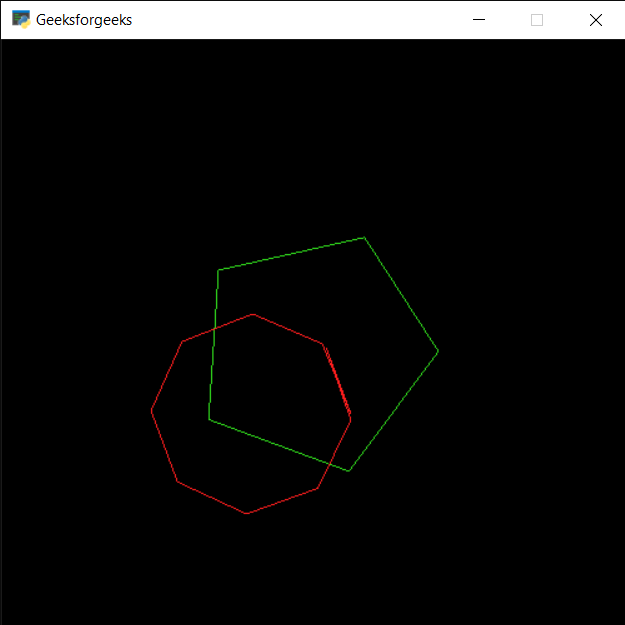# PYGLET – Drawing Arc

• Last Updated : 09 Dec, 2021

In this article we will see how we can draw arc on window in PYGLET module in python. Pyglet is easy to use but powerful library for developing visually rich GUI applications like games, multimedia etc. A window is a “heavyweight” object occupying operating system resources. Windows may appear as floating regions or can be set to fill an entire screen (fullscreen). An arc is a portion of the circumference of a circle. In the figure above, the arc is the blue part of the circle. Strictly speaking, an arc could be a portion of some other curved shape, such as an ellipse, but it almost always refers to a circle. Arc is drawn with the help of shapes module in pyglet.
We can create a window with the help of command given below

```# creating a window
window = pyglet.window.Window(width, height, title)```

In order to create window we use Arc method with pyglet.shapes
Syntax : shapes.Arc(arc_x, arc_y, size_arc, segments, angle, color, batch=batch)
Argument : It takes first two integer i.e arc position, third integer as size, forth is integer i.e segments, fifth is float i.e angle, sixth is color and last is batch object
Return : It returns Arc object

Below is the implementation

## Python3

 `# importing pyglet module``import` `pyglet` `# importing shapes from the pyglet``from` `pyglet ``import` `shapes` `# width of window``width ``=` `500``  ` `# height of window``height ``=` `500``  ` `# caption i.e title of the window``title ``=` `"Geeksforgeeks"``  ` `# creating a window``window ``=` `pyglet.window.Window(width, height, title)` `# creating a batch object``batch ``=` `pyglet.graphics.Batch()`  `# properties of circle``# co-ordinates of circle``arc_x ``=` `250``arc_y ``=` `250` `# size of arch``size_arc ``=` `100` `# segments``segments ``=` `5` `# angle``angle ``=` `20` `# color = green``color ``=` `(``50``, ``225``, ``30``)` `# creating a arc``arc1 ``=` `shapes.Arc(arc_x, arc_y, size_arc, segments, angle, color, batch ``=` `batch)` `# changing opacity of the arc1``# opacity is visibility (0 = invisible, 255 means visible)``arc1.opacity ``=` `250`  `# creating another circle with other properties``# new position = circle1_position - 50``# new size = previous radius -20``# new color = red``color ``=` `(``255``, ``30``, ``30``)` `# increase segments``segments ``=` `10` `# decreasing angle``angle ``=` `7` `# creating another arc``arc2 ``=` `shapes.Arc(arc_x``-``50``, arc_y``-``50``, size_arc``-``20``, segments, angle, color, batch ``=` `batch)` `# changing opacity of the arce2``arc2.opacity ``=` `255` `# window draw event``@window``.event``def` `on_draw():``    ` `    ``# clear the window``    ``window.clear()``    ` `    ``# draw the batch``    ``batch.draw()` `# run the pyglet application``pyglet.app.run()`

Output :My Personal Notes arrow_drop_up